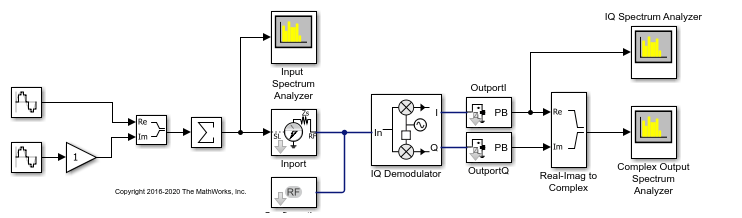## Demodulate Two-Tone RF Signal Using IQ Demodulator

Use the IQ Demodulator block to demodulate a two-tone RF signal to DC level. Observe the impairments in the demodulated output signal such as images due to gain imbalance, intermodulation distortion, and output third-order intercept (OIP3).

The two tones are at 10MHz and 15 MHz. The power of each tone is -30 dBm. The carrier frequency is 2 GHz.

### IQ Demodulator

The IQ Demodulator parameters are:

• Available power gain: 10 dB

• Local oscillator frequency: 2 GHz

• I/Q gain mismatch: 0.1 dB

• LO to RF Isolation: 90 dB

• Noise Figure: 6 dBm/Hz

Open the model.

```open('model_IQdemod') ```Run the model and observe the spectrum analyzers.

### Input Spectrum AnalyzerIn the input spectrum analyzer, you see the input RF signal with the two tones at 10 MHz and 15 MHz. The power level of each tone is -30 dBm. The carrier frequency is 2 GHz.

### I/Q Spectrum AnalyzerIn the I/Q spectrum analyzer, you see the inphase part of the demodulated signal including the DC signal level and the two tones. The following formula gives you the DC power level of the signal:

``
``

The output power level of the two tones are -20 dBm. You also see the OIP3 value (measured by the spectrum analyzer) at approximately 20 dBm.

### Complex Output Spectrum AnalyzerIn the complex output spectrum analyzer, you see the whole demodulated signal including the imaginary parts. The output power level of the two tones (10 MHz and 15 MHz) is -17 dBm.

### Image Rejection Ratio

The images of the two tones are at -10 MHz and -15 MHz. The output power level of the images are -61.78 dBm. Image rejection ratio is given by the formula:

``
``
``
``